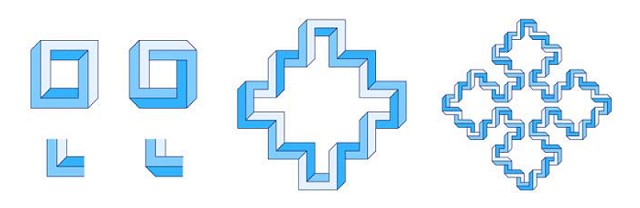# Cameron Browne. Impossible fractals

## 5. Squares

Figure 7 (left) shows two impossible multibar square designs known as four-bars or Penrose Squares, as they are obvious extensions of the tri-bar or Penrose Triangle. The four-bar on the left can be constructed using alternating sharp and truncated generators (shown underneath) while the four-bar on the right requires only the truncated generator for its construction.Figure 7. Four-bar designs (with sharp and truncated generators) applied to the square Sierpinski curve.

Figure 7 (middle) shows the development of the left four-bar as one generation of the square Sierpinski curve, and Figure 7 (right) shows the development of the right four-bar as two generations of the square Sierpinski curve. Just as the four-bar is less striking an illusion than the tri-bar, so the impossible square Sierpinski figures are less striking than the impossible snowflake and gasket figures.